# Fighting Overfitting in Deep Learning

This post outlines an attack plan for fighting overfitting in neural networks.

By ActiveWizards### Problem

While training the model, we want to get the best possible result according to the chosen metric. And at the same time we want to keep a similar result on the new data. The cruel truth is that we can’t get 100% accuracy. And even if we did, the result is still not without errors. There are simply too few test situations to find them. You may ask, what is the matter?

There are 2 types of errors: reducible and irreducible. Irreducible errors arise due to a lack of data. For example, not only the genre, duration, actors but also the mood of the person and the atmosphere while watching the film affects the rating. But we can't predict the mood of the person in the future. The other reason is the quality of the data.

Our goal is to reduce the reducible errors, which in turn are divided into Bias and Variance.

### Bias

Bias arises when we try to describe a complex process with a simple model, and as a result an error occurs. For example, it is impossible to describe a nonlinear interaction by a linear function.

### Variance

Variance describes the robust of the forecast or how much the forecast will change when the data changes. Ideally, with small changes in the data, the forecast also changes slightly.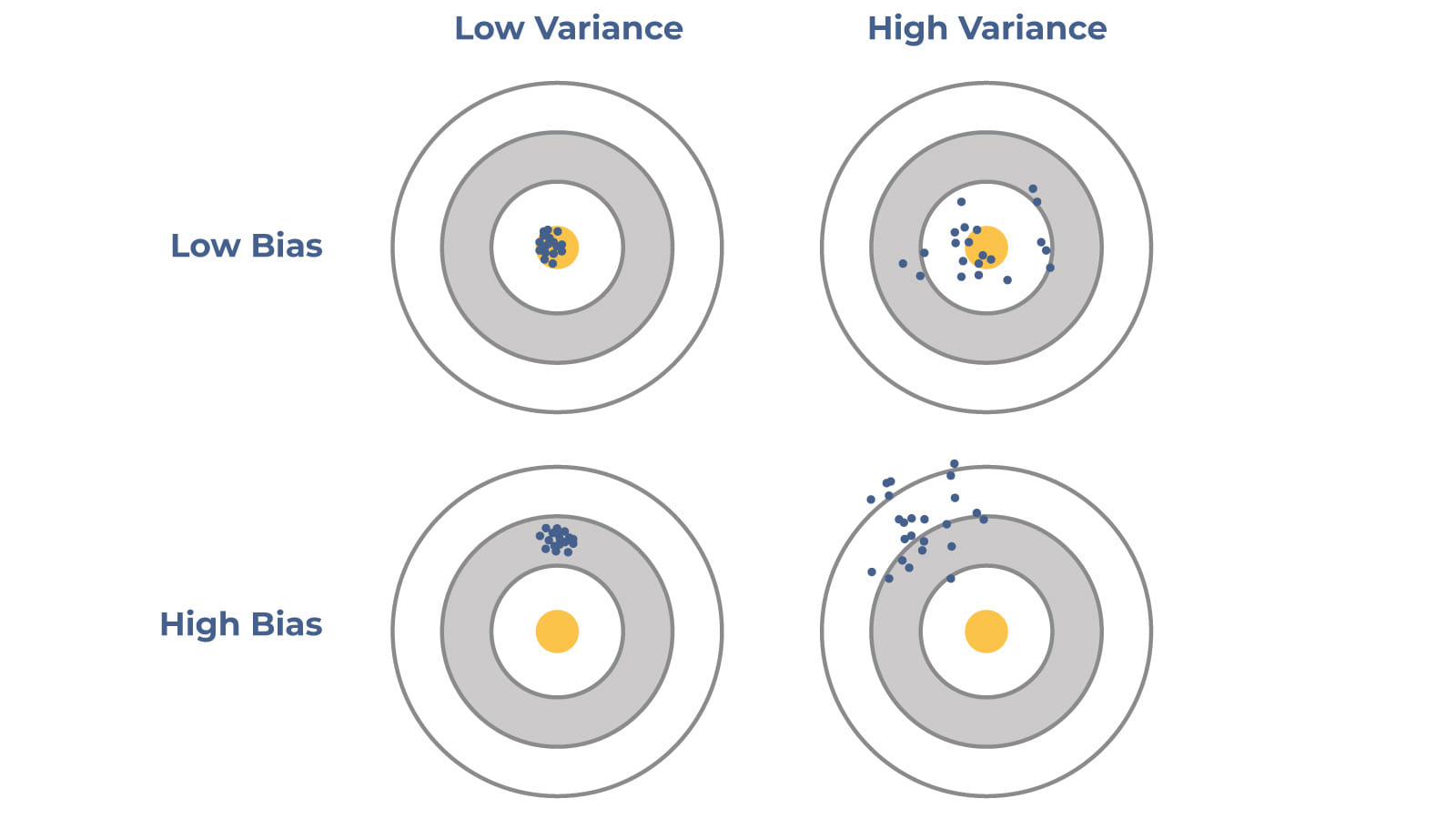The effect of Bias and Variance on the prediction

These errors are interrelated so that a decrease in one leads to an increase in the other. This issue is known as the Bias-Variance tradeoff.

The more complex (flexible) is the model (better explains the variance of the target variable), the less Bias is. But, nevertheless, the more complex is the model, the better it adapts to the training data, increasing Variance. At some point, the model will begin to find random patterns that are not repeated on new data, thereby reducing the ability of the model to generalize and increasing the error on the test data.

There is an image, describing Bias-Variance tradeoff. The red line is loss function (e.g. MSE - mean square error). The blue line is Bias and orange is Variance. As you can see, the best solution will be placed somewhere in the lines cross.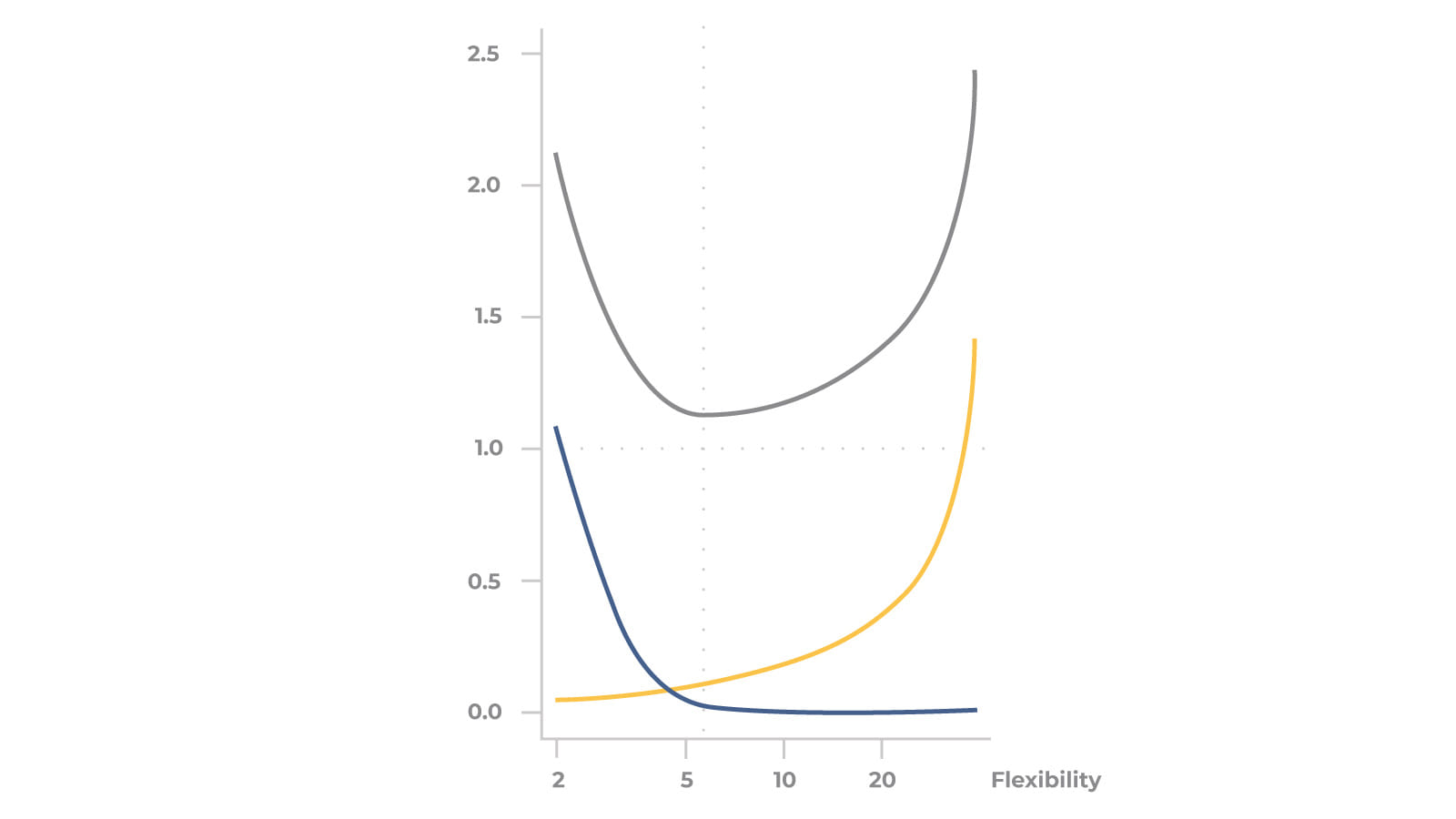Decomposition of the error function

You also can recognize that the moment when loss begins to grow is the moment when the model is overfitted.

As a result: we need to control error, to prevent not only overfitting but also underfitting.

### How can we fix this?

Regularization is a technique that discourages learning a more complex or flexible model, so as to avoid the risk of overfitting.

Note:

The problem is that model with the best accuracy in train data does not provide any guarantees, that the same model will be the best in the test data. As a result, we can choose hyperparameters with cross-validation.

### RegularizationOr, in  other words:

Cost function = Loss + Regularization term

Where Loss function is usually a function defined on a data point, prediction, and label, and measures the penalty. The Cost function is usually more general. It might be a sum of loss functions over your training set plus some model complexity penalty (regularization term).

Often an overfitted model has large weights with different signs. So that in total they level each other getting the result. The way we can improve the score is to reduce weight.

Each neuron can be represented aswhere f is an activation function, w is weight and X is data.

The “length” of the coefficient vector can be described by such a concept as the norm of the vector. And to control how much we will reduce the length of the coefficients (penalty the model for complexity and thereby prevent it from overfitting or adjusting too much to the data), we will weigh the norm by multiplying it by λ. There are no analytical methods on how to choose λ, so you can choose it by grid-search.

L2 norm (L2 regularization, Ridge)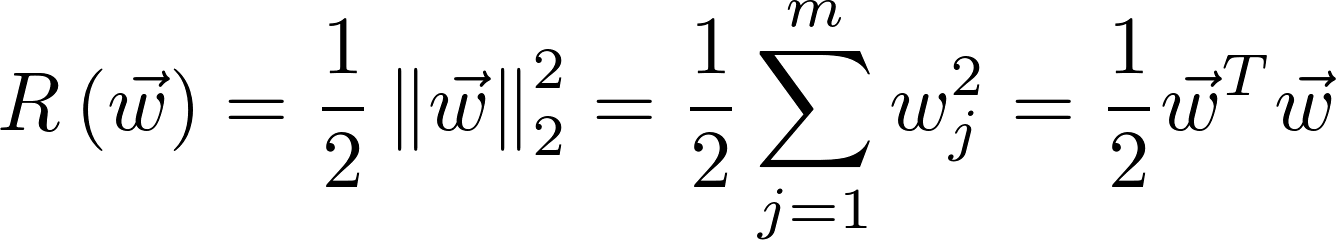If the loss is MSE, then cost function with L2 norm can be solved analytically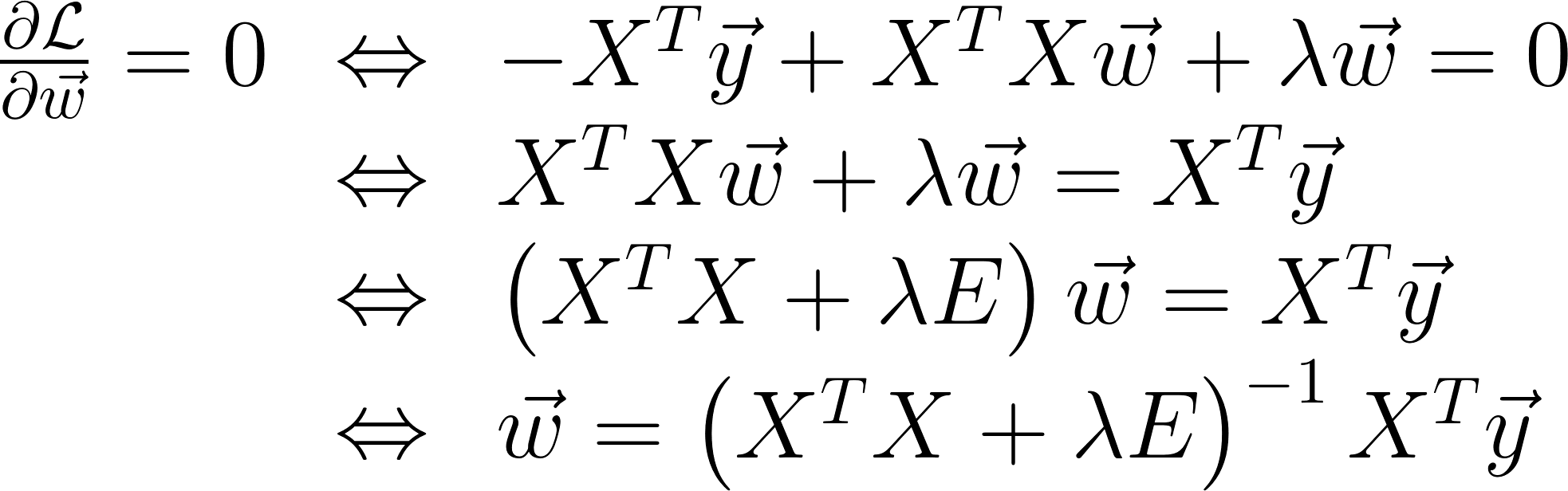There you can see that we just add an eye matrix (ridge) multiplied by λ in order to obtain a non-singular matrix and increase the convergence of the problem.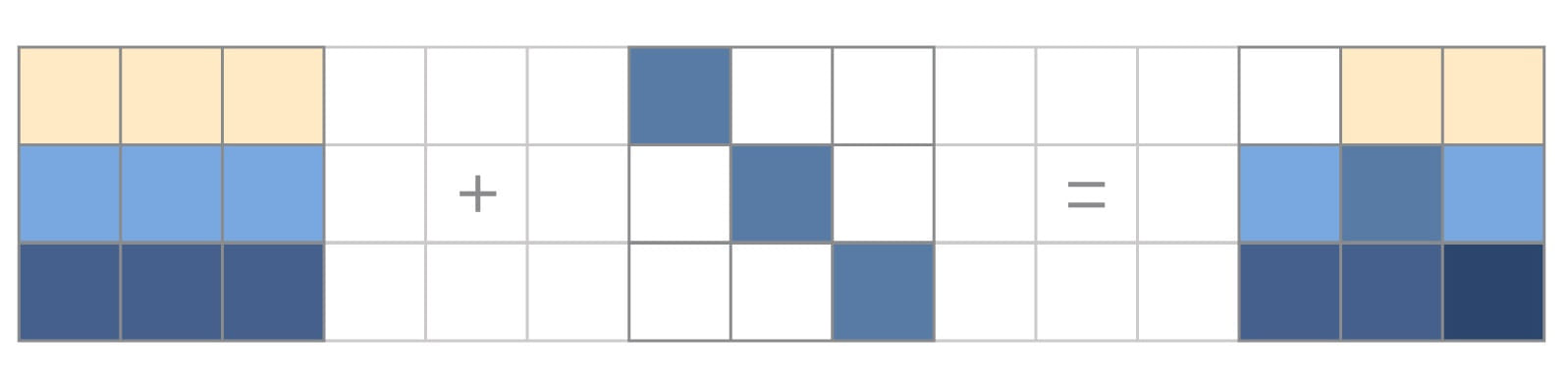Turning multicollinear XTX matrix to nonsingular one by adding an eye-matrix.

In other words, we need to minimize the cost function, so we minimize losses and the vector rate (in this case, this is the sum of the squared weights). So, the weights will be closer to zero.

Let’s calculate:

The wT is a vector-row of weights.

Before regularization: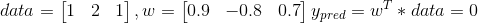Little change in data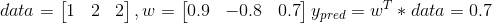After regularization, we will have the same result on test data,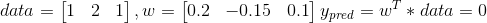But, little change in data will cause little change in result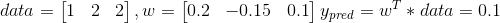Example

```from keras import regularizers
...
lambda = 0.01
kernel_regularizer=regularizers.l2(lambda)))```

L1 norm (L1 regularization, Lasso)

L1 norm means that we use absolute values of weights but not squared. There is no analytical approach to solve this.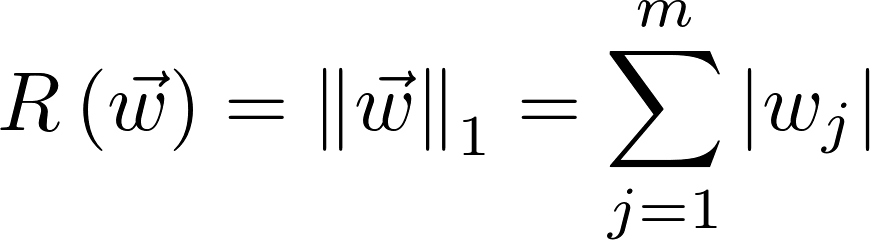This type of regression equates some weights to zero. It is very useful when we are trying to compress our model.

Example

```from keras import regularizers
...
lambda = 0.01
kernel_regularizer=regularizers.l1(lambda)))```

Let’s take a simple loss function with 2 arguments (B1 and B2) and draw a 3d plot.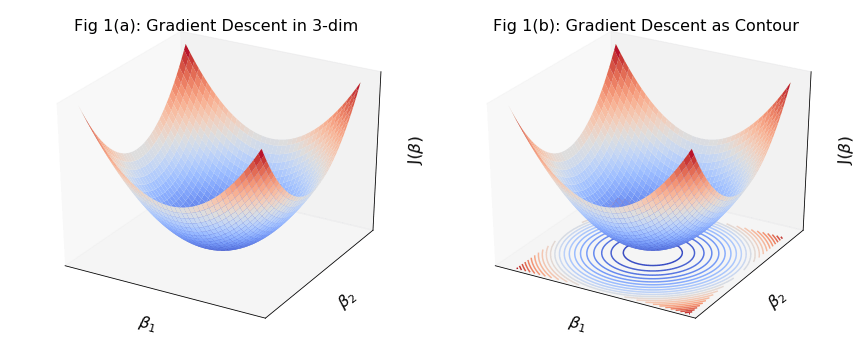Gradient descent in 3-dim with contour representation | Credits

As you can see, loss function can have the same values with different arguments. Let’s project this on arguments surface, where each point on the same curve will have the same function value. This line called the level line.

Let’s take a Lambda = 1. L2 regularization for 2 arguments function is B12 + B22 that is a circle on the plot. L2 regularization is |B1|+ |B2|, which is diamond on the plot.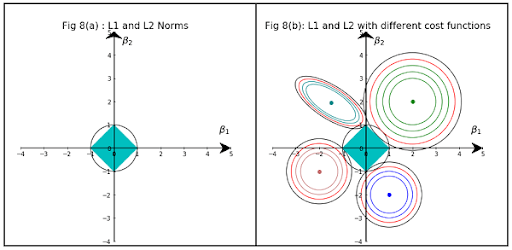L1 and L2 norms with different cost functions | Credits

Now, let’s draw different loss functions and a blue diamond (L1) and black circle (L2) regularization terms (where Lambda = 1). The truth is that the cost function will be minimum in the interception point of the red circle and the black regularization curve for L2 and in the interception of blue diamond with the level curve for L1.

### Dropouts

Imagine a simple network. For example, the development team at the hackathon. There are more experienced or smarter developers who pull the whole development on themselves, while others only help a little. If all this continues, experienced developers will become more experienced, and the rest will hardly be trained. So it is with neurons.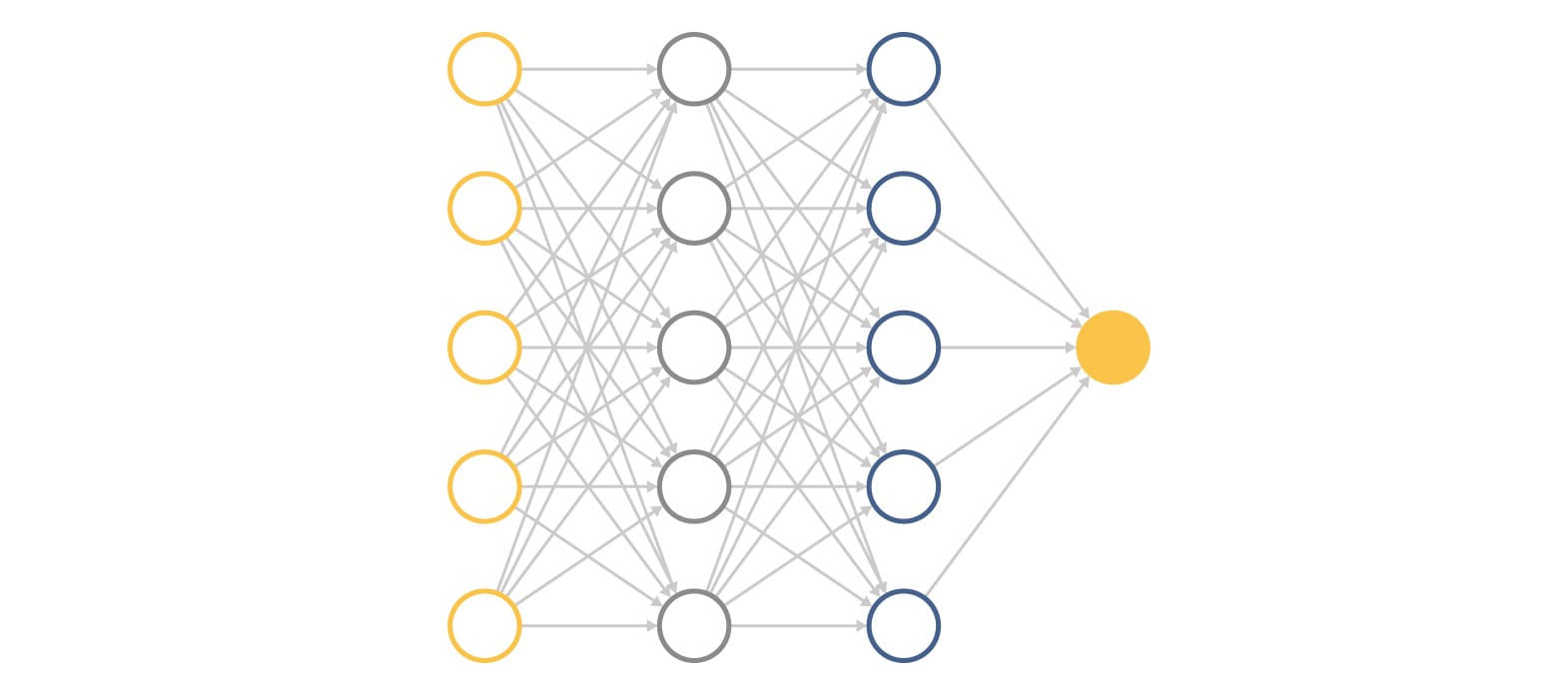Fully Connected Network

But imagine that we randomly disconnect some part of the developers at each iteration of product development. Then the rest have to turn on more and everyone learns much better.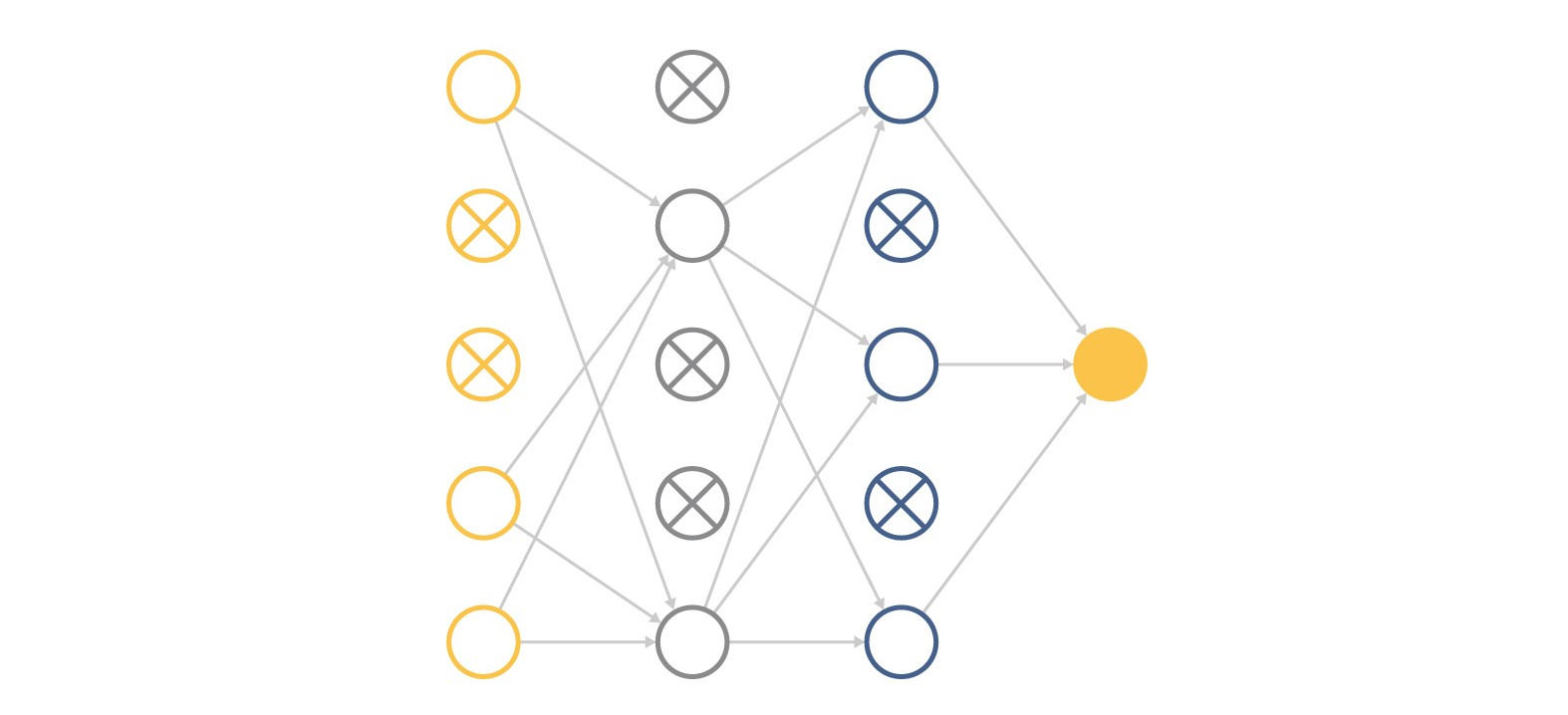Network with randomly dropped nodes

What could go wrong? If you turn off too many neurons, then remaining neurons simply will not be able to cope with their work and the result will only get worse.

It can also be thought of as an ensemble technique in machine learning. Remember, that the ensemble of strong-learners performs better than a single model as they capture more randomness and less prone to overfitting. But ensemble of weak-learners more prone to retraining than the original model.

As a result, dropout takes place only with huge neural networks.

Example

```from keras.layers.core import Dropout
…
percent_of_dropped_neurons = 0.25
model = Sequential([
Dense(32, activation='relu', input_shape=(10,)),
Dropout(percent_of_dropped_neurons)
Dense(32, activation='relu',),
Dense(1, activation='linear')
])```

### Data augmentation

The simplest way to reduce overfitting is to increase the size of the training data. In machine learning, it is hard to increase the data amount because of the high cost.

But, what about image processing? In this case, there are a few ways of increasing the size of the training data – rotating the image, flipping, scaling, shifting, etc. We also could add images without the target class to learn the network how to differentiate target from noise.

Example

```from keras.preprocessing.image import ImageDataGenerator
datagen = ImageDataGenerator(
rotation_range=40,
width_shift_range=0.2,
height_shift_range=0.2,
shear_range=0.2,
zoom_range=0.2,
horizontal_flip=True,
fill_mode='nearest')
x = img_to_array(img)              # turn image to array and reshape
x = x.reshape((1,) + x.shape)
i = 0
# the .flow() command below generates batches of randomly transformed images
# and saves the results to the `test_data_augmentation` directory
for batch in datagen.flow(x, batch_size=1,
save_to_dir='test_data_augmentation', save_prefix='data', save_format='jpeg'):
i += 1
if i > 20:
break   # generate 20 images and stop after```

Original image:Augmented images:### Early stopping

Early stopping is a kind of cross-validation strategy where we keep one part of the training set as the validation set. When we see that the performance on the validation set is getting worse, we immediately stop the training on the model.

Example

```from keras.callbacks import EarlyStopping

es = EarlyStopping(monitor='val_loss', mode='min')```

That is all we need for the simplest form of early stopping. Training will stop when the chosen performance measure stops improving. To discover the training epoch on which training was stopped, the “verbose” argument can be set to 1.

Often, the first sign of no further improvement may not be the best time to stop training. This is because the model may coast into a plateau of no improvement or even get slightly worse before getting much better.

We can account for this by adding a delay to the trigger in terms of the number of epochs on which we would like to see no improvement. This can be done by setting the “patience” argument.

`es = EarlyStopping(monitor='val_loss', mode='min', verbose=1, patience=50)`

The exact amount of patience will vary between models and problems.

By default, any change in the performance measure, no matter how fractional, will be considered an improvement. You may want to consider an improvement that is a specific increment, such as 1 unit for mean squared error or 1% for accuracy. This can be specified via the “min_delta” argument.

`es = EarlyStopping(monitor='val_accuracy', mode='max', min_delta=1)`

EarlyStopping callback can be applied with other callbacks, such as tensorboard.

```model.fit(
X_train,
y_train,
epochs=2000,
batch_size=32,
validation_data=(X_val, y_val),
callbacks=[get_tensorboard_callback('baseline_pca(45)_log', True), es])```

It is also possible to attach Tensorboard and monitor changes manually.  Every 5 minutes model will be saved into logs dir, so you can always check out to a better version.

### Neural network architecture

It is well-known that large enough networks of depth 2 can already approximate any continuous target function on [0,1]d to arbitrary accuracy (Cybenko,1989; Hornik, 1991). On the other hand, it has long been evident that deeper networks tend to perform better than shallow ones.

The recent analysis showed that “depth – even if increased by 1 – can be exponentially more valuable than width for standard feedforward neural networks”.
You can think that each new layer extracts a new feature, so that increases a non-linearity.

Remember that, increasing the depth means your model is more complex and the optimization function may not be able to find the optimal set of weights.

Image, that you have a neural network with 2 hidden layers of 5 neurons on each layer. Height = 2, width = 5. Let’s add one neuron per layer and calc a number of connections: (5+1)*(5+1) = 36 connections. Now, let’s add a new layer to the original network and calc connections: 5*5*5 = 125 connections. So, each layer will significantly increase the number of connections and execution time.

But, at the same time, this comes with the cost of increasing the chance of overfitting.

Very wide neural networks are good at memorizing data, so you shouldn't build very wide networks too. Try to build as small network, as possible, to solve your problem. Remember that the larger and complex the network is, the higher is a chance of overfitting.

You can find more here.

### Transfer learning

Why should we always start from scratch? Let’s take pre-trained models' weights and just optimize them for our task. The problem is that we haven’t a model pre-trained on our data. But we can use a network, pre-trained on a really huge amount of data. Then, we use our relatively small data to fine-tune the pre-trained model. The common practice is to freeze all layers except the last few from training.

The main advantage of transfer learning is that it mitigates the problem of insufficient training data. As you can remember, this is one of the reasons for overfitting.

Transfer learning only works in deep learning if the model features learned from the first task are general. It’s very popular to use a pre-trained model for image processing (here) and text processing, e.g. google word2vec

Another benefit is that transfer learning increases productivity and reduce training time: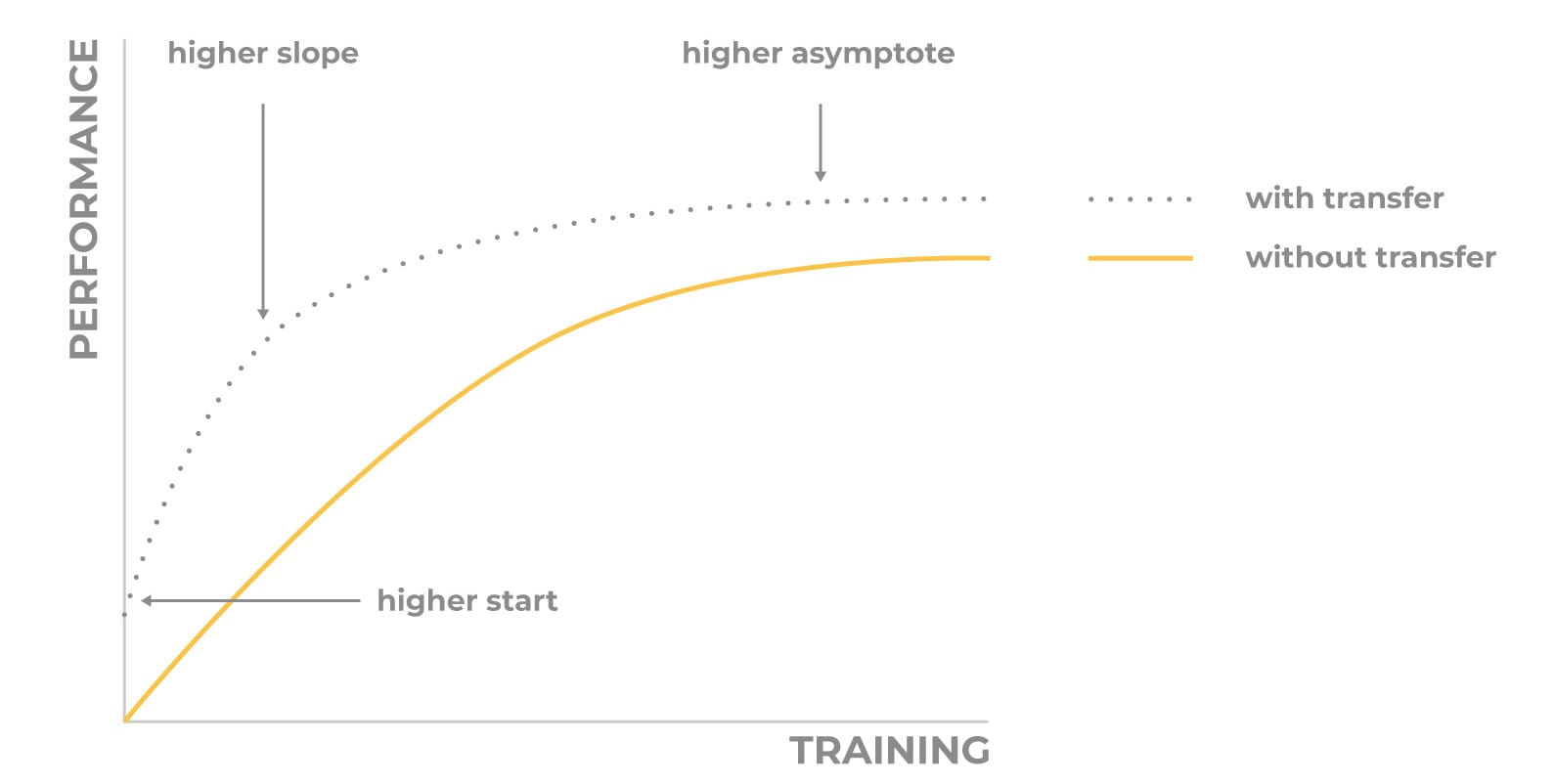Metrics function

### Batch normalization

Batch normalization allows us to not only work as a regularizer but also reduce training time by increasing a learning rate. The problem is that during a training process the distribution on each layer is changed. So we need to reduce the learning rate that slows our gradient descent optimization. But, if we will apply a normalization for each training mini-batch, then we can increase the learning rate and find a minimum faster.

Example

```from keras.layers.normalization import BatchNormalization
...
model = Sequential([
Dense(32, activation='relu', input_shape=(10,)),
BatchNormalization(),
Dense(32, activation='relu'),
Dense(1, activation='linear')
])```

### Other

There are some other less popular methods of fighting the overfitting in deep neural networks. It is not necessary that they will work. But if you have tried all other approaches and want to experiment with something else, you can read more about them here: small batch sizenoise in weights.

### Conclusion

Overfitting appears when we have a too complicated model. Our model begins to recognize noisy or random relations, that will never appear again in the new data.

One of the characteristics of this condition is large weights of different signs in neurons. There is a direct solution to this issue known as L1 and L2 regularization that can be applied to each layer separately.

The other way is to apply dropouts to the large neural network or to increase a data amount for example by data augmentation. You can also configure an early stopping callback, that will detect a moment when the model becomes overfitted.

Also, try to build such a small neural network, as possible. Choose depth and width carefully.

Don't forget that you can always use a pre-trained model and increase model productivity. At least, you can apply batch normalization to increase the learning rate and decrease overfitting at the same time.

Different combinations of these methods will give you a result and allow to solve your task.

ActiveWizards is a team of data scientists and engineers, focused exclusively on data projects (big data, data science, machine learning, data visualizations). Areas of core expertise include data science (research, machine learning algorithms, visualizations and engineering), data visualizations ( d3.js, Tableau and other), big data engineering (Hadoop, Spark, Kafka, Cassandra, HBase, MongoDB and other), and data intensive web applications development (RESTful APIs, Flask, Django, Meteor).

Original. Reposted with permission.

Related: